TM0 Surface Wave

July 17, 2017

It is well known (Stratton 1941, Collin 1991) that the interface between 2 semi-infinite regions with at least one region having finite loss associated with either a finite conductivity or equivalently an imaginary component of the dielectric constant supports an interface guided wave or Zenneck wave. The wave is a TM0 transverse magnetic mode and is characterized by the field amplitude decaying exponentially into both media away from the interface. The greater the medium loss, the less the field penetrates into the lossy medium. This surface wave mode has no cutoff wavelength and continues to propogate at arbitrarily low frequency. It is similar to the fundamental "principal" axially symmetric wire wave mode for an isolated cylindrical conductor. A general 2 dimensional interface between 2 semi-infinite media is shown below. In the general case, both media are lossy which can be described by either a finite conductivity and real refractive index or equivalentally using media complex refractive indices n1, n2 (or complex dielectric constants). In the geometry shown below, the mode propogation direction is along the z axis and the x axis is normal to the region interface and the magnetic field vector Hy is in the y direction. The electric field vector has components in both x and z directions: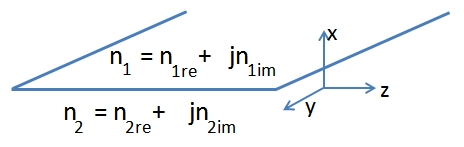Dielectric Constant, Conductivity and Refractive Index

Using the convention for the time factor below and using SI units, the bulk material propogation constant k for a time harmonic plane wave EM field at angular frequency ω = 2πf, dielectric constant ε and refractive index n for a general medium are all complex:there the values for the constants vacuum permeability μ0, permittivity ε0 and speed of light c are all exact. For a lossy medium, the imaginary parts of the material refractive index n and dielectric constant ε/ε0 are both negative (nim >0 and εim >0 in the expressions above). Depending on the wavelength/frequency being considered, separation of the material properties into an "inner" complex permittivity εi associated with bound charge and a conductivity σ associated with free charge may be artificial. At sufficiently high frequency the conductivity σ parameter associated with free charge will become complex due to the finite carrier scattering time compared to the optical period (Ramo 1984). In any case, the net response of any homogeneous material to a time harmonic EM field at a specific frequency can always be described by a complex dielectric constant or a complex refractive index.

TM0 Surface Wave

The transverse magnetic field of the TM0 surface wave is characterized by a complex modal propogation constant β along the z direction, or equivalently by a complex "mode" refractive index nm:where the field dependence normal to the interface in the x direction is:and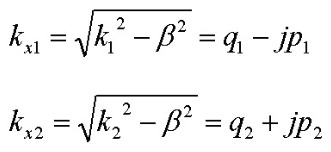and the roots are chosen so that both p1 and p2 are positive for a wave solution f(x) that attenuates in both +/- x directions away from the interface. The characteristic attenuation distances of the surface wave in the two media are:For this TM0 mode, the electric field components in the two regions are: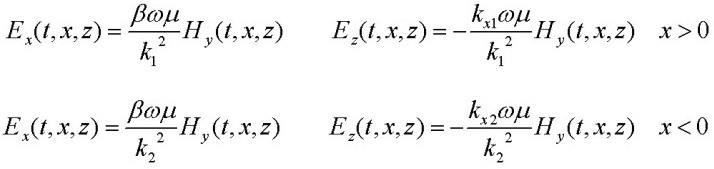and the axial z component of the power flow Poynting vector is given by: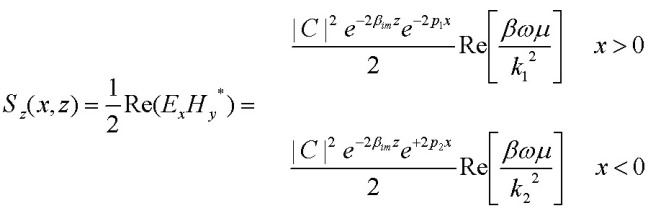Integrating over x on either side of the interface provides the total power per unit width of y carried by the surface wave in each region:The power flow across the interface at x=0, which is continuous (Hy and Ez are both continuous) is given by:For an ideal LOSSLESS dielectric region 2, and a lossy region 1, the attenuation of the power carried in the lossless dielectric is provided by a power flow across the interface into lossy region 1. This is a simple application of Poynting's Theorem (Stratton 1941) for isotropic media in region 2 for energy conservation using a thin rectangular region V of thickness δz and extending from the interface to x= - ∞ in the dielectric.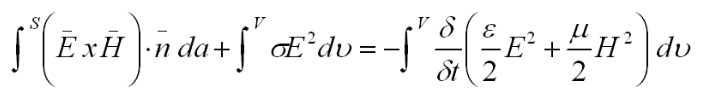The time-averaged Poynting vector flux through the bounding rectangular surface S must be zero (since the time-averaged energy density doesn't change in steady state, and there is no conversion to heat in region 2 since σ=0 in region 2) :

This flux condition leads immediately to the power attenuation rate 2βim being equal to the ratio of the power flow normal to the interface (Sx) divided by the total integrated (over x) power per unit width carried in the lossless dielectric region:which, because k2 is real, is exactly equivalent to: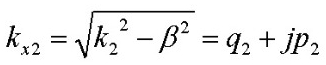Note that in the general Poynting theorem expression above, loss in a medium can be described as either a real conductivity σ or alternately as a complex medium refractive index or equivalently a complex dielectric constant ε = n^2. Note that a complex dielectric constant means that the time-averaging of the energy density term εE(t)^2 for a steady-state harmonic time dependence of the fields will lead to a non-zero value for the rate of change of energy density which represents loss. This is exactly equivalent to the result using a real conductivity value to describe the loss. Therefore either approach may be used to specify media absorption loss. The equivalency is:TM0 Propogation Constant β and Mode Index nm

In the general case with arbitrary n1 and n2, interface matching of tangential E and H fields leads to an expression (Stratton 1941), analogous to the zero reflectance Brewster angle solution for real media, for the complex propogation constant β in terms of the bulk complex propogation constants k1, k2 or complex refractice indices n1, n2 of the two regions (the magnetic permeabilities μ are assumed identical for the regions) :The corresponding TM0 surface wave "mode index", which is symmetric in interchange of n1 and n2 is:and the power attenuation of the surface wave in the z propogation direction is:(With the sign convention of Hy(x,z) above, βim and nm_im will be negative values for lossy media).

Ideal Dielectric/Conductor TM0 Surface Wave

If medium 1 is an ideal dielectric and medium 2 is a good conductor, simpler expressions for the propogation constant and surface wave attenuation are obtained.

For good conductors (at frequencies low enough that εi and σ are real) in which the conduction current is much larger than the displacement current, the real and imaginary parts of the bulk propogation constant k for the conductor (or effective complex refractive index) are equal:where εi is the bound charge dielectric constant for the conductor and δ is the skin depth for plane wave propogation.

With medium 1 having a real dielectric constant ε, the TM0 surface wave propogation constant is approximately (Stratton 1941):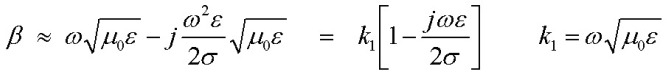and the phase velocity of the TM0 mode along the z direction is approximately equal to the velocity of light in dielectric medium 1. Properties for this ideal interface are calculated below :
 f (Hz) ε/εo εi/εo      σ (mhos/m) σ/(ωεi) k_re(m-1) n_re δ (m) β (m-1) k1 (m-1) loss (dB/m)

General TM0 Calculator

The calculator below can be used to compute the TM0 surface wave mode index and loss for arbitrary dielectric constant and conductivity of either medium. To facilitate use over a broad range of frequencies where material parameters are specified and measured differently, two different computations are provided. Units are SI (MKS):
• Using input data from first row: vacuum wavelength λ0 (m) and complex media refractive indices n1, n2
• Using input data from second row: frequency f and complex media "inner" dielectric constants ε1, ε2 (these are ε/ε0) and conductivities σ1, σ2 (mhos/m)
The first method is more useful at extremely high frequencies (infrared, optical etc.) while the second method is most useful at frequencies below ~ 100 GHz where conductivities of metals are real to a good approximation. Both methods calculate and display the results in the lower 3 lines in MKS units. The first row displays the propogation constant ko = 2π/λ and the transverse propogation constants kx1, kx2 (m-1). The second row displays the mode propogation constant β (m-1), the corresponding mode index n_m, the fractions P1, P2 of the total time-averaged power flow in the + z direction integrated over all x in the two regions, and the time-averaged power flow (except for a scaling constant) normal to the interface in the +x direction Sx1 = Re(kx1/n1^2) and Sx2 = Re(kx2/n2^2). This energy flux across the interface must be continuous so Sx1, Sx2 should be identical in value and sign which is equivalent to Ez being continuous at the interface. Note that if there is a negative power flow component (depending on the material indices), the fractional total power flow will be >1 in one region and will be negative (power flow in -z direction) in the other region with the sum equaling 1.0. The final row displays the mode power attenuation coefficient α (m-1) and corresponding dB/m value and the Hy field attenuation distances δ1, δ2 (m) in the x direction normal to the interface. When the first method is used, f and ε1, ε2 are calculated and displayed in the second row taking σ1=σ2 = 0 (a negative imaginary part of the dielectric constant is equivalent to a real conductivity). When the second method is used, the corresponding values of λ and equivalent complex material indices n1, n2 are calculated and displayed in the first row.

TM0 Surface Wave Calculator
λ (m)      n1_re     n1_im      n2_re     n2_im
f (Hz)      ε1_re     ε1_im     σ1   ε2_re     ε2_im     σ2
ko   kx1   kx2
β   n_m   P1      P2
α(m-1)   loss(dB/m)   δ1           δ2

Examples

Optical Example
The expressions above for β and nm are exact and can be applied at any electromagnetic wavelength (frequency) provided the media "constants" (complex refractive indices, dielectrics constants, conductivities) are known at that frequency. The material properties are typically frequency dependent and vary widely depending on the frequency range of interest. This example refers to an optical waveguide (Adams 1981) for which the first calculator method above is appropriate. The parameters describe an interface between metallic silver (n1) and a nonconducting glass-like dielectric (n2) at a visible wavelength with the following complex indices:
```  λ  =  0.633 µm
n1 =  0.068 -j4.0
n2 =  1.52 +j0.0
```

The TM0 surface wave mode index is:
```  nm  = 1.643127 - j0.004711
```
and the surface wave attenuation is:
```  α = 935.2 cm-1
Loss = 4062 dB/cm
```
Note that the real part of the TM0 mode index, 1.643, is greater than the real dielectric mode index,1.520, so the phase velocity of the TM0 surface wave is lower than the phase velocity of a plane wave in that dielectric medium.

The magnetic field amplitude for this example is shown below:The second example uses parameters in the short-wave radio frequency range. A 30 MHz surface wave propogates over an ideal flat salt-water body. In this case the appropriate material parameters are the low frequency real dielectric constant ~ 81 and typical salt-water conductivity ~ 4 mhos/m. These "low frequency" parameters are fairly constant in the frequency range from DC to ~ 1 GHz. Using the calculator above with the second method, the TMO surface wave mode index is:
```  nm  = 0.999993  -j0.000208
```
and the surface wave travels along the air/water interface at very nearly the speed of light in air. The surface wave attenuation is:
```  α = .262 km-1
Loss = 1.14 dB/km
```
The TM0 radio wave exponential penetration depth into the water is ~ 4.7 cm and above the water into air is ~ 112 m. At this frequency, salt water is a good conductor since σ/(εω) ~ 30.

The graph below shows the TM0 wave field penetration into air and typical salt water, and the propogation loss versus frequency over a range where the dielectric constant and conductivity are approximately constant. For salt water the "good conductor" condition σ/(ωε) >>1 is valid below ~ 300 MHz. In this region, the surface wave loss increases as the square of the frequency:Attenuation and Field Penetration vs. Conductivity

The graph below shows the penetration depth and attenuation at f = 10 MHz as a function of conductivity for the TM0 surface wave in the ideal case of an interface between a perfect dielectric with ε/εo = 1.0 and a conducting medium with variable conductivity σ and a dielectric constant of 1.0. In the higher conductivity region σ > 0.1 mho/m, the mode attenuation decreases linearly with conductivity and the penetration depth into the conductor decreases with the square root of the conductivity (same as a pure plane wave propogating in a good conductor) while the field penetration into the dielectric medium increases as the square root of the conductivity of the conducting medium:From Cylindrical Wire Wave to Planar Surface Wave

It is interesting to consider the propogation loss of the principal symmetric TM0 wire wave mode for a long cylindrical straight conducting wire and the limit as the wire radius becomes infinitely large which should transition to the TM0 planar surface wave discussed above. The wire wave solution with cylindrical symmetry is considerably more complex than that for the 2 dimensional planar geometry. Assuming the displacement current is negligible compared for the conduction current in the wire and the external medium is lossless with a real dielectric constant ε and bulk propogation constant k2, the complex propogation constant β (or the corresponding transverse propogation constant in the external dielectric h) is given by the solution of the transcendental equation below:The "principal" solution is the solution with β close to k2 and for or any reasonable wire radius, ha<<1 leading to a simple iterative method (Sommerfeld 1952) for solving the above equation for h and β . However, transitioning to the infinite wire radius requires that ha> 1 and determining intermediate behaviour requires numerical solution of the transcendental equation. The graph belows shows the computed propogation loss for the copper wire wave solution for f = 10 MHz over a wide range or radius showing that the solution of the transcendental equation converges to the loss result expected for the planar surface wave case. The asymptotic loss value corresponds to the result predicted above for the case of a good conductor and a dielectric planar interface:Reference:
• Electromagnetic Theory, J. Stratton, 1941, McGraw Hill, pp. 517-524
• Field Theory of Guided Waves, R. E. Collin, 1991, IEEE Press, pp.697-700
• Fields and Waves in Communication Electronics, S. Ramo, J. Whinnery, T. Van Duzer, 1984, J. Wiley & Sons. pp. 279-283
• An Introduction To Optical Waveguides, M. J. Adams, 1981, J. Wiley & Sons, pp 66-68
• Electrodynamics, A. Sommerfeld, Lectures on Theoretical Physics, 1952, VIII Academic Press, pp 188-185